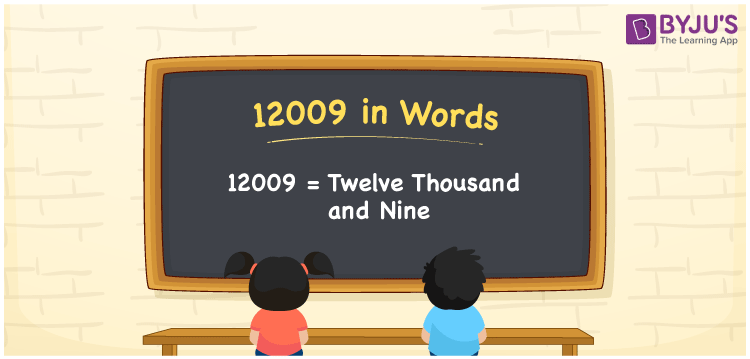# 12009 in Words

The number 12009 in words is “Twelve thousand and nine”. If you purchased a cycle worth Rs. 12009, you can write it as “I purchased a cycle worth Rs. Twelve thousand and nine. Also, the number 12009 is a natural number that lies between 12008 and 12010. Now, let us learn how to write the number 12009 in words using the place value system.

 12009 in Words: Twelve Thousand and Nine. Twelve Thousand and Nine in Numerical Form: 12009.

## 12009 in English Words## How to Write 12009 in Words?

The place value table for the numeral 12009 is provided below up to 5 digits.

 Ten-thousands Thousands Hundreds Tens Ones 1 2 0 0 9

The expanded form of 12009 is as follows:

= 1 × 10000 + 2 × Thousand + 0 × Hundred + 0 × Ten + 9 × One

= 1 × 10000 + 2 × 1000 + 0 × 100 + 0 × 10 + 9 × 1

= 10000 + 2000 + 9

= 12009

= Twelve thousand and nine

Hence, 12009 in words is twelve thousand and nine.

12009 in words – Twelve thousand and nine

Is 12009 an odd number? – Yes

Is 12009 an even number? – No

Is 12009 a perfect square number? – No

Is 12009 a perfect cube number? – No

Is 12009 a prime number? – No

Is 12009 a composite number? – Yes

## Frequently Asked Questions on 12009 in Words

Q1

### How to spell 12009 in words?

12009 in words is twelve thousand and nine.

Q2

### Simplify 12000 + 9, and express it in words.

Simplifying 12000 + 9, we get 12009. Hence, 12009 in words is twelve thousand and nine.

Q3

### Is 12009 an even number?

No, 12009 is not an even number.